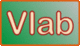online_compound_interest_1 calculator

 r' = 0.01 r A = P[1 + (r'/n)]nt P = Initial investment amount (USD) r = Interest rate, annual (%) t = Length of time the money is invested (years) n = Number of times the interest is compounded per year A = Total investment payback amount (USD)INPUT:
Initial investment amount P (USD) :

Interest rate r, annual (%):

Length of time t the money is invested (years) :

Number of times n the interest is compounded per year :
 1  (Yearly)  12  (Monthly)  365  (Daily)

 OUTPUT:

n =

Total investment payback amount A :   0.00

Request processed at  07:50:58 am on July 5th, 2022  [ 220705  07:50:58 ].

Thank you for running online_compound_interest_1